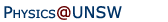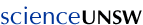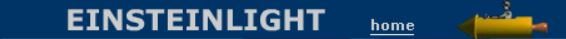Relativity in brief... or in detail..

## Electricity and magnetism in a moving frame: an electric circuit example

If you accepted what we said in An introduction to the mechanics of Galileo and Newton, or better still if you did some experiments yourself to check what we said, then you will probably be convinced that doing mechanics experiments on a smoothly moving ship, train or plane will give you the same results that you get on the ground.

Now let's take an explicit example. An LC (inductance-capacitance) circuit is often used as an electric oscillating circuit or a filter, so it can be used to measure frequency and time.

 A parallel plate capacitor in vacuum creates an electric field between two plates proportional to the charge stored on it. Put charge q on one plate and -q on the other, and the voltage difference V is q/C, where C, the capacitance, is given bywhere A is the plate area and d the separation and ε0 is the permittivity of free space. (This equation requires a numerical factor unless d2 is negligible compared to A) For a long coil with length D, cross section a, and N turns, the inductance L is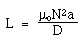where μ0 is the magnetic permeability of free space. The resonant frequency of this circuit is easily found (see AC resonance) to be: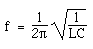. Before we go further, does the resonant frequency of this circuit change when you take it on the train? If so, why? Substituting gives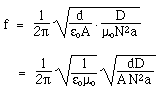where the first square root term is one we have seen before in The electric and magnetic forces between moving charges. It is just c, the speed of light. So the resonant frequency of the circuit depends on the speed of light and on geometrical properties of the coil and the capacitor, for reasons suggested in the previous link. Substituting, we obtain:So, if this circuit behaves the same on the train and on the platform, what do we expect of the speed of light? And if the circuit behaves differently, why? If you are in the mood, revise AC circuits.A resonant circuitHome | Summary | Quiz | Credits School of Physics - The University of New South Wales Sydney, Australia 2052 © School of Physics UNSW 2052 Disclaimer feedback